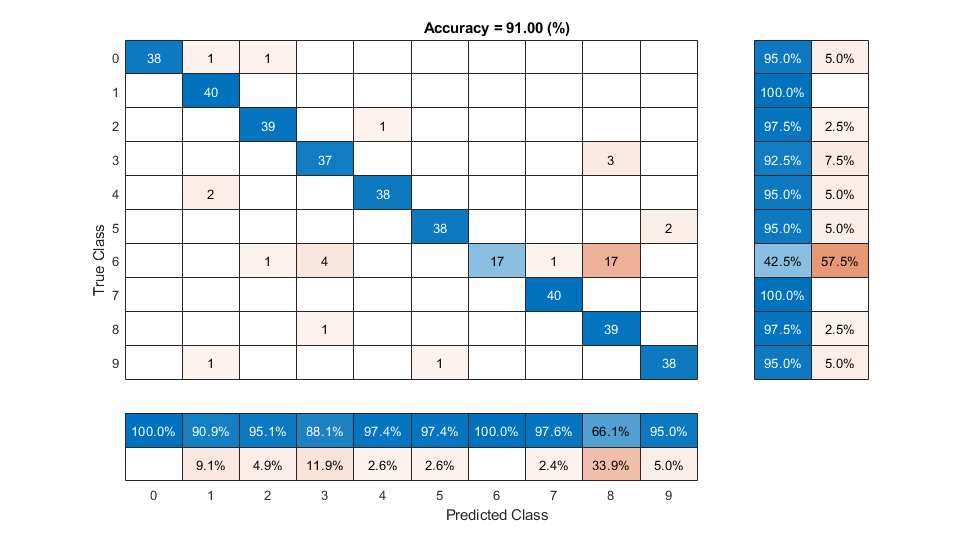# ivector

Extract i-vector

## Syntax

``w = ivector(ivs,data)``
``w = ivector(___,'ApplyProjectionMatrix',TF)``

## Description

example

````w = ivector(ivs,data)` extracts i-vectors from the input `data`.```
````w = ivector(___,'ApplyProjectionMatrix',TF)` specifies whether to apply the projection matrix determined using `trainClassifier`.```

## Examples

collapse all

An i-vector system consists of a trainable front end that learns how to extract i-vectors based on unlabeled data, and a trainable backend that learns how to classify i-vectors based on labeled data. In this example, you apply an i-vector system to the task of word recognition. First, evaluate the accuracy of the i-vector system using the classifiers included in a traditional i-vector system: probabilistic linear discriminant analysis (PLDA) and cosine similarity scoring (CSS). Next, evaluate the accuracy of the system if you replace the classifier with bidirectional long short-term memory (BiLSTM) network or a K-nearest neighbors classifier.

Create Training and Validation Sets

Download the Free Spoken Digit Dataset (FSDD) . FSDD consists of short audio files with spoken digits (0-9).

```url = "https://ssd.mathworks.com/supportfiles/audio/FSDD.zip"; downloadFolder = tempdir; datasetFolder = fullfile(downloadFolder,'FSDD'); if ~exist(datasetFolder,'dir') fprintf('Downloading Free Spoken Digit Dataset ...\n') unzip(url,datasetFolder) end```

Create an `audioDatastore` to point to the recordings. Get the sample rate of the data set.

```ads = audioDatastore(datasetFolder,'IncludeSubfolders',true); [~,adsInfo] = read(ads); fs = adsInfo.SampleRate;```

The first element of the file names is the digit spoken in the file. Get the first element of the file names, convert them to categorical, and then set the `Labels` property of the `audioDatastore`.

```[~,filenames] = cellfun(@(x)fileparts(x),ads.Files,'UniformOutput',false); ads.Labels = categorical(string(cellfun(@(x)x(1),filenames)));```

To split the datastore into a development set and a validation set, use `splitEachLabel`. Allocate 80% of the data for development and the remaining 20% for validation.

`[adsTrain,adsValidation] = splitEachLabel(ads,0.8);`

Create an i-vector system that expects audio input at a sample rate of 8 kHz and does not perform speech detection.

`wordRecognizer = ivectorSystem('DetectSpeech',false,"SampleRate",fs)`
```wordRecognizer = ivectorSystem with properties: InputType: 'audio' SampleRate: 8000 DetectSpeech: 0 EnrolledLabels: [0×2 table] ```

Train the i-vector extractor using the data in the training set.

```trainExtractor(wordRecognizer,adsTrain, ... "UBMNumComponents",512, ... "UBMNumIterations",5, ... ... "TVSRank",128, ... "TVSNumIterations",3);```
```Calculating standardization factors ....done. Training universal background model ........done. Training total variability space ...done. i-vector extractor training complete. ```

Train the i-vector classifier using the data in the training data set and the corresponding labels.

```trainClassifier(wordRecognizer,adsTrain,adsTrain.Labels, ... "NumEigenvectors",16, ... ... "PLDANumDimensions",16, ... "PLDANumIterations",3);```
```Extracting i-vectors ...done. Training projection matrix .....done. Training PLDA model ......done. i-vector classifier training complete. ```

Enroll labels into the system using the entire training set.

`enroll(wordRecognizer,adsTrain,adsTrain.Labels)`
```Extracting i-vectors ...done. Enrolling i-vectors .............done. Enrollment complete. ```

In a loop, read audio from the validation datastore, identify the most-likely word present according to the specified scorer, and save the prediction for analysis.

```trueLabels = adsValidation.Labels; predictedLabels = trueLabels; reset(adsValidation) scorer ="plda"; for ii = 1:numel(trueLabels) audioIn = read(adsValidation); to = identify(wordRecognizer,audioIn,scorer); predictedLabels(ii) = to.Label(1); end```

Display a confusion chart of the i-vector system's performance on the validation set.

```figure('Units','normalized','Position',[0.2 0.2 0.5 0.5]) confusionchart(trueLabels,predictedLabels, ... 'ColumnSummary','column-normalized', ... 'RowSummary','row-normalized', ... 'Title',sprintf('Accuracy = %0.2f (%%)',100*mean(predictedLabels==trueLabels)))```Evaluate Deep Learning Backend Performance

Next, train a fully-connected network using i-vectors as input.

```ivectorsTrain = (ivector(wordRecognizer,adsTrain))'; ivectorsValidation = (ivector(wordRecognizer,adsValidation))';```

Define a fully-connected network.

```layers = [ ... featureInputLayer(size(ivectorsTrain,2),'Normalization',"none") fullyConnectedLayer(128) dropoutLayer(0.4) fullyConnectedLayer(256) dropoutLayer(0.4) fullyConnectedLayer(256) dropoutLayer(0.4) fullyConnectedLayer(128) dropoutLayer(0.4) fullyConnectedLayer(numel(unique(adsTrain.Labels))) softmaxLayer classificationLayer];```

Define training parameters.

```miniBatchSize = 256; validationFrequency = floor(numel(adsTrain.Labels)/miniBatchSize); options = trainingOptions("adam", ... "MaxEpochs",10, ... "MiniBatchSize",miniBatchSize, ... "Plots","training-progress", ... "Verbose",false, ... "Shuffle","every-epoch", ... "ValidationData",{ivectorsValidation,adsValidation.Labels}, ... "ValidationFrequency",validationFrequency);```

Train the network.

`net = trainNetwork(ivectorsTrain,adsTrain.Labels,layers,options);`Evaluate the performance of the deep learning backend using a confusion chart.

```predictedLabels = classify(net,ivectorsValidation); trueLabels = adsValidation.Labels; figure('Units','normalized','Position',[0.2 0.2 0.5 0.5]) confusionchart(trueLabels,predictedLabels, ... 'ColumnSummary','column-normalized', ... 'RowSummary','row-normalized', ... 'Title',sprintf('Accuracy = %0.2f (%%)',100*mean(predictedLabels==trueLabels)))```Evaluate KNN Backend Performance

Train and evaluate i-vectors with a k-nearest neighbor (KNN) backend.

Use `fitcknn` to train a KNN model.

```classificationKNN = fitcknn(... ivectorsTrain, ... adsTrain.Labels, ... 'Distance','Euclidean', ... 'Exponent',[], ... 'NumNeighbors',10, ... 'DistanceWeight','SquaredInverse', ... 'Standardize',true, ... 'ClassNames',unique(adsTrain.Labels));```

Evaluate the KNN backend.

```predictedLabels = predict(classificationKNN,ivectorsValidation); trueLabels = adsValidation.Labels; figure('Units','normalized','Position',[0.2 0.2 0.5 0.5]) confusionchart(trueLabels,predictedLabels, ... 'ColumnSummary','column-normalized', ... 'RowSummary','row-normalized', ... 'Title',sprintf('Accuracy = %0.2f (%%)',100*mean(predictedLabels==trueLabels)))```References

 Jakobovski. "Jakobovski/Free-Spoken-Digit-Dataset." GitHub, May 30, 2019. https://github.com/Jakobovski/free-spoken-digit-dataset.

## Input Arguments

collapse all

i-vector system, specified as an object of type `ivectorSystem`.

Data to transform, specified as a cell array or as an `audioDatastore`, `signalDatastore`, or `TransformedDatastore` object.

• If InputType is set to `'audio'` when the i-vector system is created, specify `data` as one of these:

• If InputType is set to `'features'` when the i-vector system is created, specify `data` as one of these:

• A matrix with underlying type `single` or `double`. The matrix must consist of audio features where the number of features (columns) is locked the first time `trainExtractor` is called and the number of hops (rows) is variable-sized. The number of features input in any subsequent calls to any of the object functions must be equal to the number of features used when calling `trainExtractor`.

• A cell array of matrices with underlying type `single` or `double`. The matrices must consist of audio features where the number of features (columns) is locked the first time `trainExtractor` is called and the number of hops (rows) is variable-sized. The number of features input in any subsequent calls to any of the object functions must be equal to the number of features used when calling `trainExtractor`.

• A `TransformedDatastore` object with an underlying `audioDatastore` or `signalDatastore` whose `read` function has output as described in the previous bullet.

• A `signalDatastore` object whose `read` function has output as described in the first bullet.

Data Types: `cell` | `audioDatastore` | `signalDatastore`

Indicates whether the linear discriminant analysis (LDA) and within-class covariance normalization (WCCN) projection matrix determined using `trainClassifier` is applied.

• If the projection matrix was trained, then `ApplyProjectionMatrix` defaults to `true`.

• If the projection matrix was not trained, then `ApplyProjectionMatrix` defaults to `false` and cannot be set to `true`.

Data Types: `logical`

## Output Arguments

collapse all

Extracted i-vectors, returned as a column vector or a matrix. The number of columns of `w` is equal to the number of input signals. The number of rows of `w` is the dimension of the i-vector.